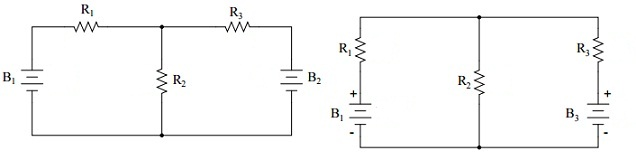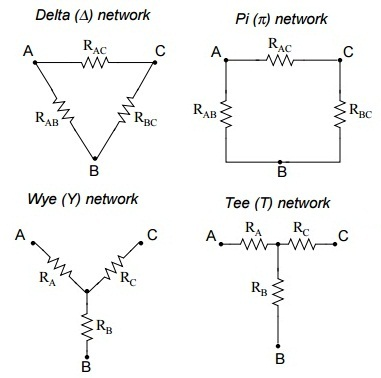#### Circuit Theorems, Physics tutorial

Introduction to Circuit Theorems:

The theorems of Electric circuit are for all time advantageous to assist in determining the current and voltage in multi loop circuits. In electric network analysis, the basic rules are Ohm's Law and Kirchhoff's Laws.

As such humble laws might be applied to examine just regarding any circuit configuration (that is, even if we have to resort to complicated algebra to handle multiple unknowns), there are several shortcut processes of analysis to make the math simpler for the average human. Such theorems utilize basic rules or formulas and fundamental equations of mathematics to examine fundamental components of electrical or electronics parameters like currents, voltages, resistance and so forth. Such basic theorems comprise the basic theorems such as Superposition theorem, Tellegen's theorem, Norton's theorem, Maximum power transfer theorem and Thevenin's theorems.

Some of the group of network theorems that are generally employed in the circuit analysis process comprises Compensation theorem, Substitution theorem, Reciprocity theorem, Millman's theorem and the Miller's theorem.

Millman's Theorem:

In Millman's Theorem, the circuit is re-sketched as a parallel network of branches, each and every branch having a resistor or series battery or resistor combination. The Millman's Theorem is applicable just to such circuits that can be re-drawn accordingly.By assuming the supply voltage in each and every branch and the resistance in each branch, Millman's Theorem will state us the voltage across all the branches. Please it is noted that we have labeled the battery in the rightmost branch as 'B3' to clearly signify it as being in the third branch, even although there is no 'B2' in the circuit!

Millman's Theorem is nothing more than a lengthy equation, applied to any circuit drawn as a set of parallel-connected branches, each and every branch having its own voltage source and series resistance:

[(EB1/R1) + (EB2/R2) + (EB3/R3)]/[(1/R1) + (1/R2) + (1/R3)]

Millman's Theorem is extremely suitable for finding out the voltage across a set of parallel branches, where there are adequate voltage sources present to preclude solution through regular series-parallel reduction process. It as well is simple in the sense that it does not need the use of concurrent equations.

Superposition Theorem:

The Super position theorem is a manner to find out the currents and voltages present in the circuit that consists of multiple sources (taking one source at a time). The super position theorem defines that in a linear network having a number of current or voltage sources and resistances, the current via any branch of the network is the arithmetical sum of the currents due to each of the sources if acting independently.

Super position theorem is employed only in the linear networks. This theorem is employed in both AC and DC circuits wherein it assists to construct Thevenin's and Norton's equivalent circuit.

The strategy utilized in the Superposition Theorem is to remove all however one source of power in a network at a time, by employing series or parallel analysis to find out the voltage drops (and/or currents) in the modified network for each and every power source separately. Then, once voltage drops and/or currents have been find out for each power source working separately, the values are all 'superimposed' on top of each other (added arithmetically) to determine the real voltage drops/currents having all sources active.

The sources can be eradicated by employing the given methodology:

a) Ideal voltage sources are short-circuited.

b) Ideal current sources are open-circuited.

Thevenin's Theorem:

Thevenin's theorem gives a helpful tool whenever resolving complex and large electric circuits by decreasing them to a single voltage source in series by a resistor. It is mainly beneficial where a single resistor or load in a circuit is subject to change.

Officially, the Thevenin's theorem can be defined as:

'Any two-terminal linear electric circuit comprising of resistors and sources, can be substituted through an equivalent circuit having a single voltage source in series having a resistor joined across the load'.

The given steps summarize the method to simplify an electric circuit by employing Thevenin's theorem where VTH and RTH are the Thevenin's voltage and Thevenin's resistance correspondingly.

• Take out the load resistance RL.
• VTH is the open circuit (OC) voltage across the load terminals.
• RTH is the resistance across the load terminals having all sources substituted via their internal resistances.

Alternatively measure the OC voltage across, and the short circuit (SC) current via the load terminals. Then,

VTH = Voc and RTH = Voc/ISC

Norton's Theorem:

Norton's Theorem defines that it is likely to simplify any linear circuit, no matter how complex, to an equivalent circuit having just a single current source and parallel resistance joined to a load. Just as by Thevenin's Theorem, the qualification of 'linear' is similar to that found in the Superposition Theorem: all fundamental equations should be linear (that is, no exponents or roots).

Thevenin's equivalent circuit is a practical voltage source. In disparity, Norton's equivalent circuit is a practical current source. This can be officially defined as:

'Any hvo-terminal, linear circuit, of resistors and sources, can be substituted via a single current source in parallel having a resistor'.

To find out the Norton's equivalent circuit, Norton current, 'IN', and Norton resistance 'RN' are needed. The given steps summarize the method needed.

Take away the load resistance, RL.

IN is the SC current via the load terminals

RN is the resistance across the load terminals having all sources substituted by their internal resistances. Obviously RN = RTH

Maximum Power Transfer Theorem:

The Maximum Power Transfer Theorem is not so much a ways of analysis as it is a help to system design. Simply defined, the maximum amount of power will be dissipated through a load resistance if that load resistance is equivalent to the Thevenin's/Norton's resistance of the network supplying the power. When the load resistance is higher or lower than the Thevenin's/Norton's resistance of the source network, its dissipated power will be less than the maximum.

This theorem describes the condition for the maximum power transfer to load under different circuit conditions. The theorem defines that the power transfer via a source to a load is maximum in a network if the load resistance is equivalent to the internal resistance of the source. For AC circuits load impedance must match having the source impedance for maximum power transfer even when the load is operating at diverse power factor.

The maximum power transfer theorem defines that the power delivered to the load is maximum if the load resistance, RL is equivalent to the internal (source) resistance, RS of the DC power supply. In another words, it can be stated that the load resistance should match the Thevenin's resistance for maximum power transfer to occur, that is,

(RS = RTH) = RL

If this takes place, the voltage across the load resistance will be VS/2 and the power delivered to the load is represented by:

P = VLIL = I2LRL

P = [VS2/(RS + RL)2] RL

Reciprocity Theorem:

Reciprocity theorem assists to determine the other corresponding solution even devoid of further work, once the circuit is examined for one solution. The theorem defines that in a linear passive bilateral network, the excitation source and its corresponding response can be substituted.

Compensation Theorem:

In any bilateral active network, when the amount of impedance is altered from the original value to some other value carrying a current of 'I', then the resultant changes which takes place in other branches are similar as those that would have been caused due to the injection voltage source in the modified branch with a negative sign, that is, minus of voltage current and changed impedance product.

Δ-Y and Y-Δ conversions:

In most of the circuit applications, we encounter components joined altogether in one of two manners to form a three-terminal network: the 'Delta', or Δ (as well termed as the 'Pi', or 'π') configuration, and the 'Y' (as well termed as the 'T') configuration.This is possible to compute the proper values of resistors essential to form one type of network (Δ or Y) which behaves identically to the other type, as examined from the terminal connections alone. That is, when we had two separate resistor networks, one Δ and one Y, each having its resistors hidden from view, with nothing however the three terminals (A, B, and C) exposed for testing, the resistors could be sized for the two networks in such a way that there would be no way to electrically find out one network apart from the other. In another words, equivalent Δ and Y networks behave identically.

There are some equations employed to change one network to the other:

To convert a delta (Δ) to a Wye (Y):

RA = (RAB RAC)/(RAB + RAC + RBC)

RB = (RAB RBC)/(RAB + RAC + RBC)

RC = (RAC RBC)/(RAB + RAC + RBC)

To convert a Wye (Y) to a delta (Δ):

RAB = (RARB + RARC + RBRC)/RC

RBC = (RARB + RARC + RBRC)/RA

RAC = (RARB + RARC + RBRC)/RB

Tutorsglobe: A way to secure high grade in your curriculum (Online Tutoring)

Expand your confidence, grow study skills and improve your grades.

Since 2009, Tutorsglobe has proactively helped millions of students to get better grades in school, college or university and score well in competitive tests with live, one-on-one online tutoring.

Using an advanced developed tutoring system providing little or no wait time, the students are connected on-demand with a tutor at www.tutorsglobe.com. Students work one-on-one, in real-time with a tutor, communicating and studying using a virtual whiteboard technology.  Scientific and mathematical notation, symbols, geometric figures, graphing and freehand drawing can be rendered quickly and easily in the advanced whiteboard.

Free to know our price and packages for online physics tutoring. Chat with us or submit request at info@tutorsglobe.com